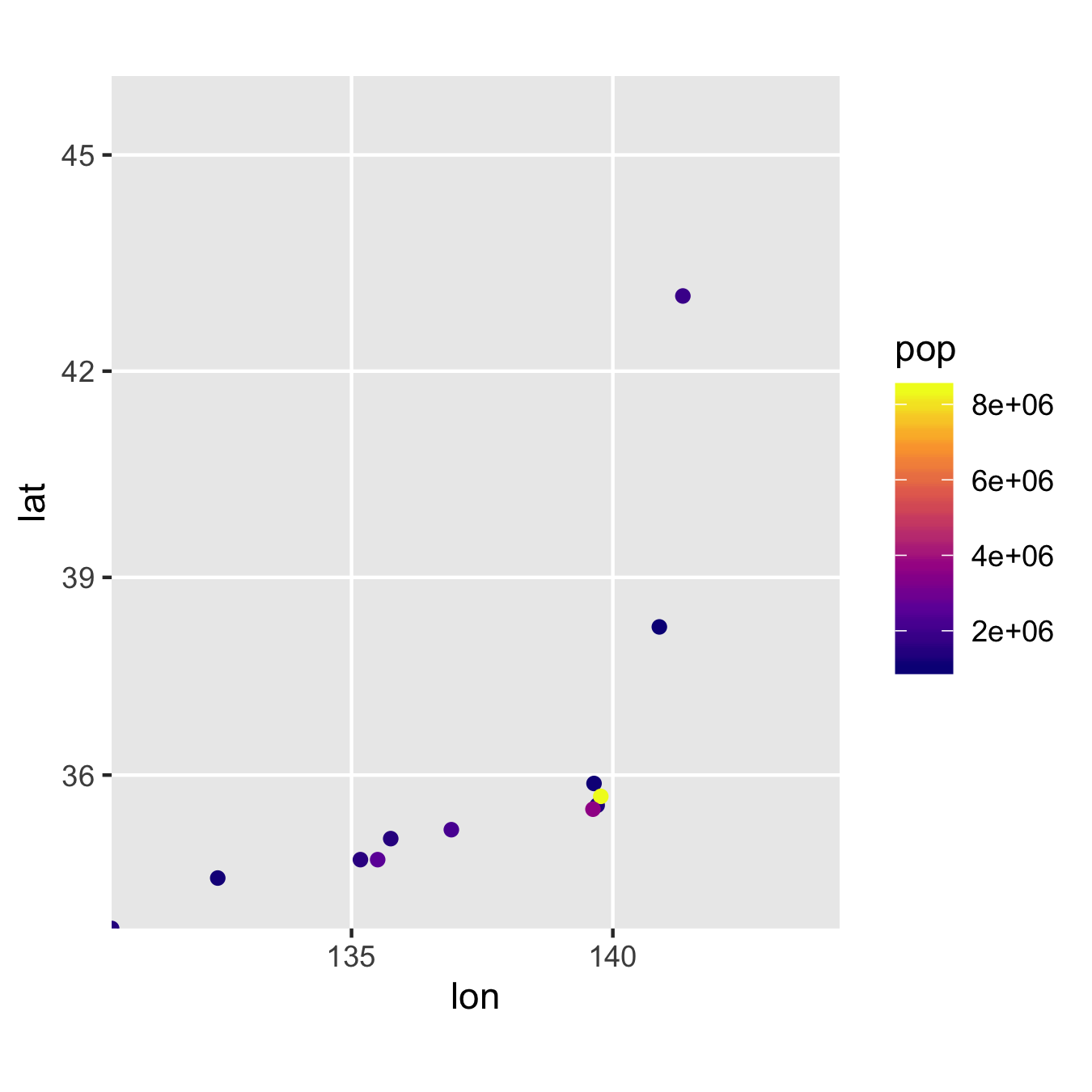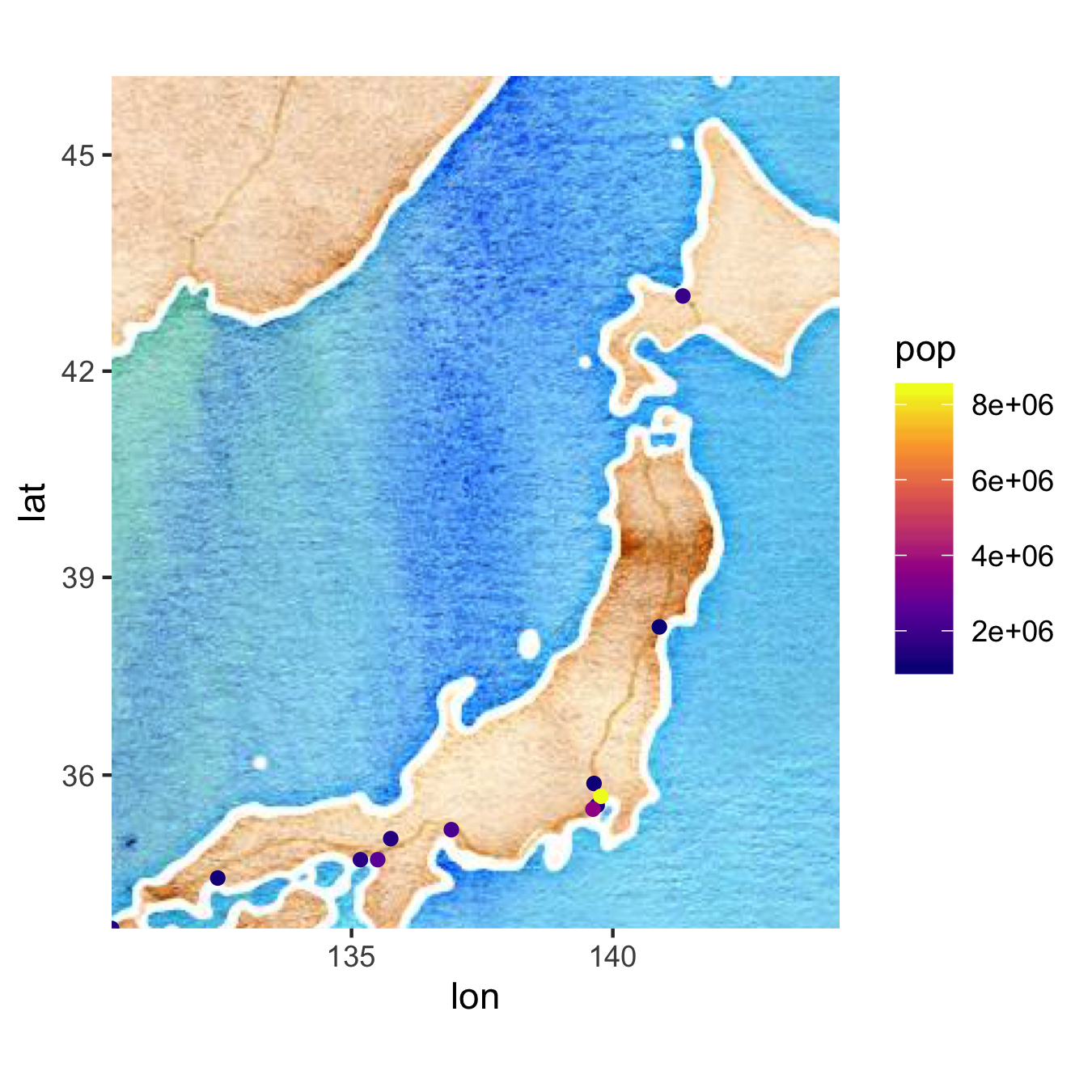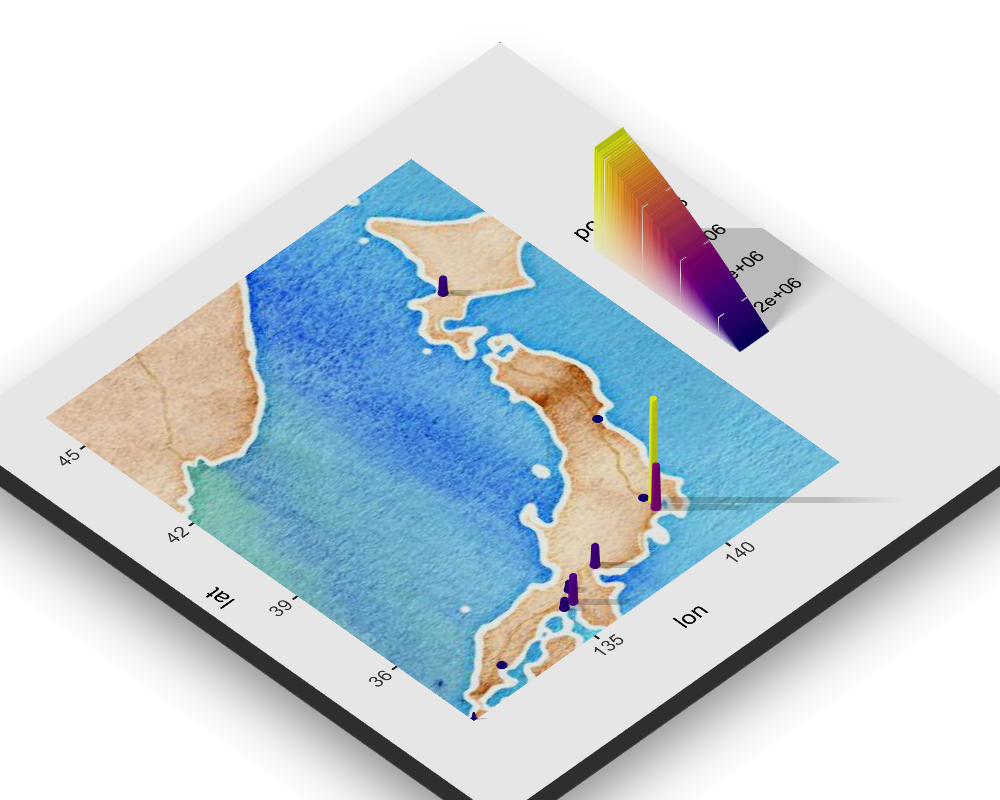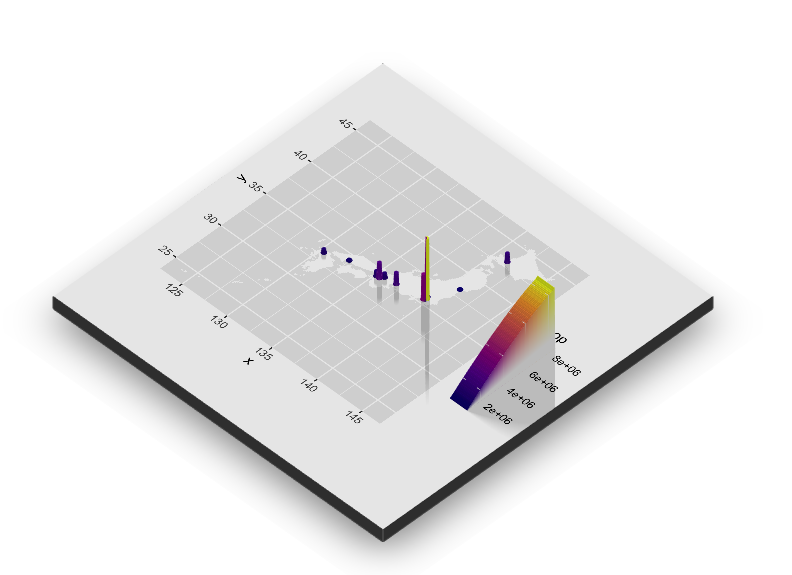Skip to content
{{ message }}

Instantly share code, notes, and snippets.

# jebyrnes/rayshade_maps_gg.R

Created Jun 27, 2019
 #Rayshader reprex to try and put a flat raster map under a #set of 3d points library(rayshader) library(ggplot2) library(dplyr) library(maps) library(ggmap) data(world.cities) japan <- world.cities %>% filter(country.etc == "Japan") %>% filter(pop > 1e6) japan_map <- get_stamenmap(bbox = c(left = min(japan\$long), bottom = min(japan\$lat), right = max(japan\$long)+3, top = max(japan\$lat)+3), maptype = "watercolor", zoom = 5) #plot together together_plot <- ggmap(japan_map) + geom_point(data = japan, aes(x = long, y = lat, color = pop)) + scale_color_viridis_c(option = "C") #Separate plots map_plot <- ggmap(japan_map) point_plot <- ggplot() + geom_point(data = japan, aes(x = long, y = lat, color = pop)) + scale_color_viridis_c(option = "C") #together plot_gg(together_plot,multicore=TRUE, width=4, height=4, scale=250, windowsize = c(1000,800)) render_camera(zoom = 0.5, theta = 130, phi = 35) render_snapshot("together.png") #Apart plot_gg(list(map_plot, point_plot),multicore=TRUE, width=4, height=4, scale=250, windowsize = c(1000,800)) render_camera(zoom = 0.5, theta = 130, phi = 35) render_snapshot("apart.png") #apart2 plot_gg(list(point_plot, map_plot),multicore=TRUE, width=4, height=4, scale=250, windowsize = c(1000,800)) render_camera(zoom = 0.5, theta = 130, phi = 35) render_snapshot("apart2.png")

###tylermorganwall commented Jun 27, 2019

 Since this uses ggmap, you have to do some manual manipulation of that object to get this to plot correctly. Here, I make the map transparent: ```library(rayshader) library(ggplot2) library(dplyr) library(maps) library(ggmap) data(world.cities) japan <- world.cities %>% filter(country.etc == "Japan") %>% filter(pop > 1e6) japan_map <- get_stamenmap(bbox = c(left = min(japan\$long), bottom = min(japan\$lat), right = max(japan\$long)+3, top = max(japan\$lat)+3), maptype = "watercolor", zoom = 5) #plot together japan_map_attributes <- attributes(japan_map) japan_map_trans <- matrix(adjustcolor(japan_map, alpha.f = 0), nrow = nrow(japan_map)) attributes(japan_map_trans) <- japan_map_attributes``` Here are the two ggplots: ```together_plot <- ggmap(japan_map_trans) + geom_point(data = japan, aes(x = long, y = lat, color = pop)) + scale_color_viridis_c(option = "C")``````point_plot <- ggmap(japan_map) + geom_point(data = japan, aes(x = long, y = lat, color = pop)) + scale_color_viridis_c(option = "C")```I use the map with a transparent ggmap layer as the height layer, and drape the full one with the stamen layer on top of it (using the list interface in `plot_gg()`). I also increased the size slightly to prevent some overlap between two points. ```plot_gg(list(point_plot,together_plot),multicore=TRUE, width=4.5, height=4.5, scale=250, windowsize = c(1000,800))```###jebyrnes commented Jun 27, 2019

 OK, cool. So the basic point is to blank out the layer you want flat in one ggplot? Let's see if this holds in general for a polygon... ``````library(sf) japan_poly <- sf::st_as_sf(map("world", plot = FALSE, fill = TRUE)) %>% filter(ID=="Japan") #A plot we want to see point_plot_poly <- ggplot() + geom_sf(data = japan_poly) + geom_point(data = japan, aes(x = long, y = lat, color = pop)) + scale_color_viridis_c(option = "C") #A plot with japan blanked out, but included together_plot_poly <- ggplot() + geom_sf(data = japan_poly, alpha = 0, color = NA, fill = NA) + geom_point(data = japan, aes(x = long, y = lat, color = pop)) + scale_color_viridis_c(option = "C") #combine! plot_gg(list(point_plot_poly,together_plot_poly),multicore=TRUE, width=4.5, height=4.5, scale=250, windowsize = c(1000,800)) `````` Note, I initially tried this with just alpha = 0, but, didn't work - really needed to blank the whole thing.

###jebyrnes commented Jun 28, 2019

 Works for rasters, too! Make a raster from the polygon ``````library(raster) library(fasterize) ref_rast <- raster(japan_poly, res = 0.1) japan_rast <- fasterize(japan_poly, raster = ref_rast) `````` Use RStoolbox to plot the raster ``````library(RStoolbox) #A plot we want to see point_plot_rast <- ggR(japan_rast) + geom_point(data = japan, aes(x = long, y = lat, color = pop)) + scale_color_viridis_c(option = "C") #A plot with japan blanked out, but included together_plot_rast <- ggR(japan_rast, alpha = 0) + geom_point(data = japan, aes(x = long, y = lat, color = pop)) + scale_color_viridis_c(option = "C") plot_gg(list(point_plot_rast,together_plot_rast),multicore=TRUE, width=4.5, height=4.5, scale=250, windowsize = c(1000,800)) ``````to join this conversation on GitHub. Already have an account? Sign in to comment